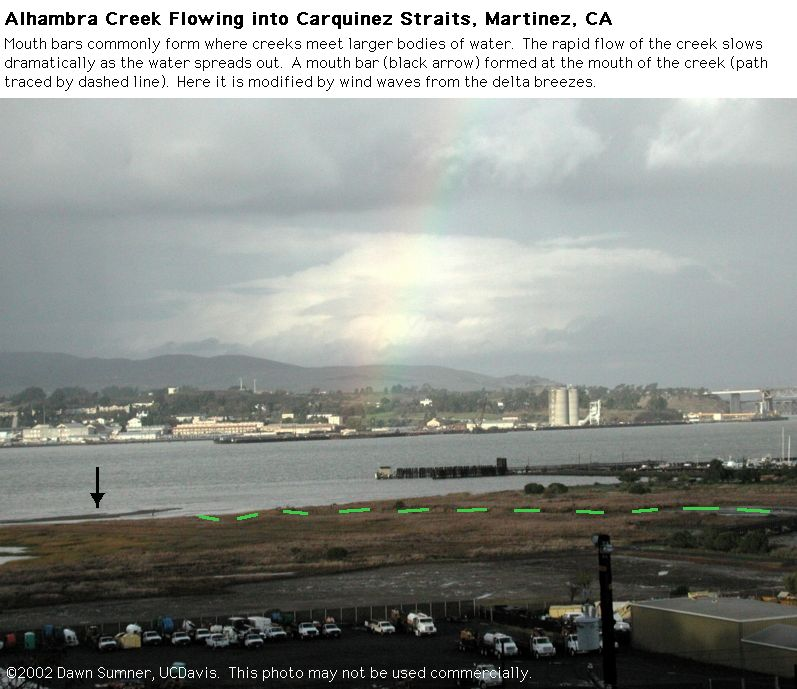# 6.1.8: Shoreline Environments (Chapter 13)

$$\newcommand{\vecs}{\overset { \rightharpoonup} {\mathbf{#1}} }$$ $$\newcommand{\vecd}{\overset{-\!-\!\rightharpoonup}{\vphantom{a}\smash {#1}}}$$$$\newcommand{\id}{\mathrm{id}}$$ $$\newcommand{\Span}{\mathrm{span}}$$ $$\newcommand{\kernel}{\mathrm{null}\,}$$ $$\newcommand{\range}{\mathrm{range}\,}$$ $$\newcommand{\RealPart}{\mathrm{Re}}$$ $$\newcommand{\ImaginaryPart}{\mathrm{Im}}$$ $$\newcommand{\Argument}{\mathrm{Arg}}$$ $$\newcommand{\norm}{\| #1 \|}$$ $$\newcommand{\inner}{\langle #1, #2 \rangle}$$ $$\newcommand{\Span}{\mathrm{span}}$$ $$\newcommand{\id}{\mathrm{id}}$$ $$\newcommand{\Span}{\mathrm{span}}$$ $$\newcommand{\kernel}{\mathrm{null}\,}$$ $$\newcommand{\range}{\mathrm{range}\,}$$ $$\newcommand{\RealPart}{\mathrm{Re}}$$ $$\newcommand{\ImaginaryPart}{\mathrm{Im}}$$ $$\newcommand{\Argument}{\mathrm{Arg}}$$ $$\newcommand{\norm}{\| #1 \|}$$ $$\newcommand{\inner}{\langle #1, #2 \rangle}$$ $$\newcommand{\Span}{\mathrm{span}}$$$$\newcommand{\AA}{\unicode[.8,0]{x212B}}$$Figure $$\PageIndex{1}$$: Mouth bar from a creek flowing into brackish water straits (California)Figure $$\PageIndex{2}$$: Mouth bar from a creek flowing into brackish water straits (California)

Large sections of the central California coast are mostly erosional with substantial cliffs.Figure $$\PageIndex{3}$$: Modern Wave Cut Platform​​​​​​​Figure $$\PageIndex{4}$$: Ancient Wave Cut Platform​​​​​​​

# Scott Creek Beach

Scott Creek Beach, CA, is a wave and storm dominated beach. The following photos were taken near low tide. We go to this beach on the second field trip.Figure $$\PageIndex{5}$$: Scott Creek Beach​​​​​​​Figure $$\PageIndex{6}$$: Terrace at Scott Creek Beach​​​​​​​Figure $$\PageIndex{7}$$: Beach StratificationFigure $$\PageIndex{8}$$: Beach StratificationFigure $$\PageIndex{9}$$: Beach StratificationFigure $$\PageIndex{10}$$: Beach Stratification

The first photo shows beach stratification along the beach, whereas the next photo shows strata deposited at about the same time, but in a terrace perpendicular to the beach. Note how the laminae appear much more parallel in the first photo. This is because the main slope of the beach is towards the ocean. When looking in the rock record, it is important to understand the 3-D geometry of deposits for the best interpretations.Figure $$\PageIndex{11}$$: Beach StratificationFigure $$\PageIndex{12}$$: Beach StratificationFigure $$\PageIndex{13}$$: Antidunes in a creek

This Brazilian beach is also wave dominatedFigure $$\PageIndex{14}$$: Modern Beach Stratification

Pescadero Beach and estuary have complex facies changes over short distances. We visit this site on the second field trip.Figure $$\PageIndex{15}$$: Pescadero BeachFigure $$\PageIndex{16}$$: The creek flows into the breaker zoneFigure $$\PageIndex{17}$$: Looking across the estuaryFigure $$\PageIndex{18}$$: Looking towards the shoreline from the estuary​​​​​​​Figure $$\PageIndex{19}$$: Dunes migrating in the creek​​​​​​​Figure $$\PageIndex{20}$$: Dunes migrating in the creek​​​​​​​Figure $$\PageIndex{21}$$: Tidal flats next to the creek channelFigure $$\PageIndex{22}$$: Ripples beside the creekFigure $$\PageIndex{23}$$: Current ripples beside the creek​​​​​​​

Figure $$\PageIndex{24}$$: Eroded sand dunes​​​​​​​

# Broome Town Beach

Broome Town Beach, Western Australia, is tide dominated, although small waves are present. In the summer, typhoons are common. The following photos were taken at low tide on the tidal flat in the winter.Figure $$\PageIndex{25}$$: Modern Wave RipplesFigure $$\PageIndex{26}$$: Modern Wave RipplesFigure $$\PageIndex{27}$$: Modern Wave Ripples

# Derby

The coastline near Derby, Western Australia, is dominated by extremely high tides (almost 12 m tidal range), and waves are essentially absent in the protected Prince William Sound. Mangroves line the shore. The following photos were taken at an exceptionally low tide when the tidal bars were exposed.Figure $$\PageIndex{28}$$: Tidal barFigure $$\PageIndex{29}$$: Tidal bar dunes

# Lake Louise

The sediment-rich creek that flows into Lake Louise, southern Canadian Rockies, creates a lacustrine delta, as seen in the following photographs.Figure $$\PageIndex{30}$$: Lacustrine DeltaFigure $$\PageIndex{31}$$: Lacustrine Delta